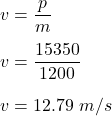## A car with a mass of 1200 kg has a momentum of 15, 350 kg * m/s. What is its velocity?

Question

A car with a mass of 1200 kg has a momentum of 15, 350 kg * m/s. What is its velocity?

in progress 0
2 weeks 2021-09-05T14:26:11+00:00 1 Answers 0 views 0

v = 12.79 m/s

Explanation:

Given that,

The mass of a car, m = 1200 kg

Momentum of the car, p = 15 350 kg-m/s

We need to find the velocity of the car. We know that, the formula for the momentum of an object is given by :

p = mv

Where

v is the velocity of the bject

So,So, the velocity of the car is 12.79 m/s.